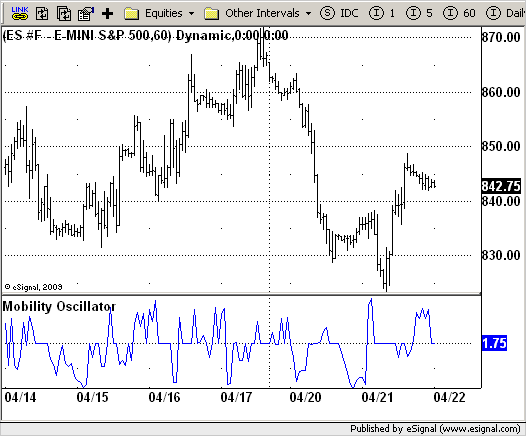# Mobility Oscillator

ICE Data Services -

MobOsc.efs
EFSLibrary - Discussion Board

File Name: MobOsc.efs

Description:
Mobility Oscillator

Formula Parameters:

• M : 10
• LookBack : 14

Notes:
The price distribution function, which analyzes the distribution of prices over a lookback period, is useful for predicting price mobility.
Here's a new method called the mobility oscillator that will allow you to do so.
Price mobility, the ease with which prices move, can be assessed by constructing price distribution functions and determining congestion and the location of the current price compared with the congestion. That is not to say that moves will happen, since other external factors not in the market influence moves, but that it is easier. When congestion develops and the current price is in a congested region, an abrupt move often follows.

MobOsc.efsEFS Code:

```/*********************************
Provided By:
eSignal (Copyright c eSignal), a division of Interactive Data
Formula Script (EFS) is for educational purposes only and may be
modified and saved under a new file name.  eSignal is not responsible
for the functionality once modified.  eSignal reserves the right
to modify and overwrite this EFS file with each new release.

Description:
Mobility Oscillator

Version:            1.0  04/21/2009

Formula Parameters:                     Default:
M                                   10
LookBack                            14
Notes:
The price distribution function, which analyzes the distribution of
prices over a lookback period, is useful for predicting price mobility.
Here's a new method called the mobility oscillator that will allow you
to do so.
Price mobility, the ease with which prices move, can be assessed by
constructing price distribution functions and determining congestion and
the location of the current price compared with the congestion. That is
not to say that moves will happen, since other external factors not in
the market influence moves, but that it is easier. When congestion develops
and the current price is in a congested region, an abrupt move often follows.

**********************************/
var fpArray = new Array();
var bInit = false;

function preMain() {
setStudyTitle("Mobility Oscillator");
setCursorLabelName("MobilityOsc");
var x = 0;
fpArray[x] = new FunctionParameter("M", FunctionParameter.NUMBER);
with(fpArray[x++]) {
setLowerLimit(1);
setDefault(10);
}
fpArray[x] = new FunctionParameter("LookBack", FunctionParameter.NUMBER);
with(fpArray[x++]) {
setLowerLimit(1);
setDefault(14);
}
}

var xMobOsc = null;

function main(M, LookBack) {
var nBarState = getBarState();
var nMobOsc = 0;
if (nBarState == BARSTATE_ALLBARS) {
if (M == null) M = 10;
if (LookBack == null) LookBack = 14;
}
if (bInit == false) {
xMobOsc = efsInternal("Calc_MobOsc", M, LookBack);
bInit = true;
}
nMobOsc = xMobOsc.getValue(0);
if (nMobOsc == null) return;
return nMobOsc;
}

var bSecondInit = false;
var xHH = null;
var xLL = null;
var xHigh = null;
var xLow = null;
var xClose = null;

function Calc_MobOsc(M, LookBack) {
var i = 0;
var j = 0;
var n = 0;
var RX = 0;
var IMX = 1;
var BU = 0;
var BL = 0;
var BUpdf = 0;
var BLpdf = 0;
var Value99 = 0;
var PDFVar = 0;
var PDF = 0;
var PDFMX = 0;
var PDFC = 0;
if (getCurrentBarCount() <= LookBack * 2 + 2) return;
if (bSecondInit == false) {
xHigh = high();
xLow = low();
xClose = close();
xHH = upperDonchian(LookBack, xHigh);
xLL = lowerDonchian(LookBack, xLow);
bSecondInit = true;
}

HMax = xHH.getValue(0);
LMin = xLL.getValue(0);
RX = (HMax - LMin) / M;

for (i = 1; i <= M; i++) {
BU = LMin + i * RX;
BL = BU - RX;
BLpdf = LMin + (i - 1) * RX;
BUpdf = LMin + i * RX;
PDFVar = 0;
for (j = 0; j < LookBack; j++) {
n = i + j;
if (xHigh.getValue( - n) <= BUpdf) PDFVar++;
if ((xHigh.getValue( - n) <= BUpdf) || (xLow.getValue( - n) >= BUpdf)) Value99 = 1;
else PDFVar = PDFVar + (BUpdf - xLow.getValue( - n)) / (xHigh.getValue( - n) - xLow.getValue( - n));
if (xHigh.getValue( - n) <= BLpdf) PDFVar--;
if ((xHigh.getValue( - n) <= BLpdf) || (xLow.getValue( - n) >= BLpdf)) Value99 = 1;
else PDFVar = PDFVar - (BLpdf - xLow.getValue( - n)) / (xHigh.getValue( - n) - xLow.getValue( - n));
}
PDF = PDFVar / LookBack;
if (i == 1) PDFMX = PDF;
if (PDF > PDFMX) {
IMX = i;
PDFMX = PDF;
}
if (i == 1) PDFC = PDF;
if ((xClose.getValue( - (LookBack - 1)) > BL) && (xClose.getValue( - (LookBack - 1)) <= BU)) PDFC = PDF;
}
var PMO = LMin + (IMX - 0.5) * RX;
var MO = 100 * (1 - PDFC / PDFMX);
if (xClose.getValue( - (LookBack - 1)) < PMO) MO = -MO;
return - MO;
}```